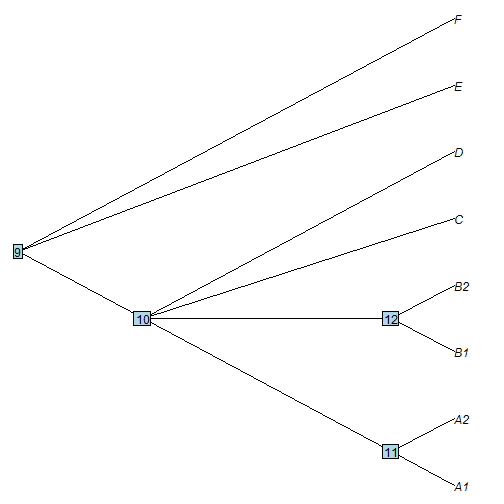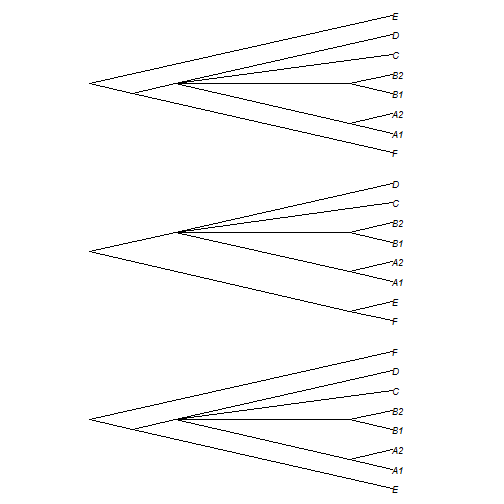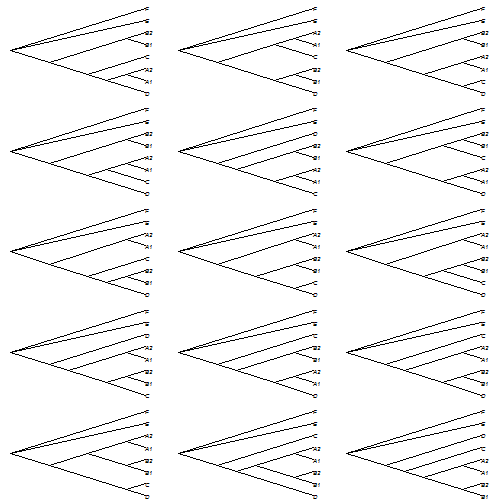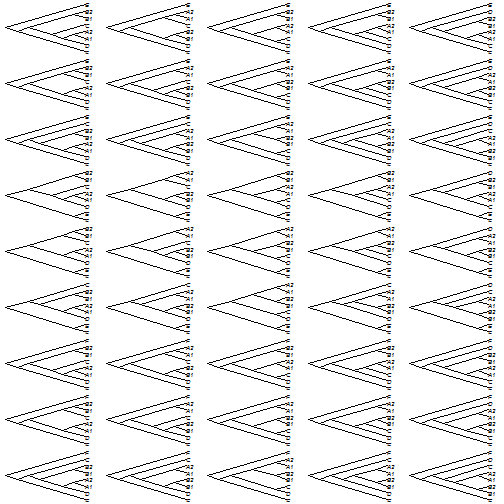## Tuesday, August 9, 2016

### Resolving one or more multifurcations in all possible ways

This may be unnecessarily complicated, but for reasons that I will address in a future post, I wanted to write some code to resolve polytomies in all possible ways.

Now, there exists a function in ape, `multi2di`, that resolves polytomies randomly and by grouping taxa in the order that they appear, but (so far as I know), no prior R function finds all possible resolutions of a polytomy.

The code chunk below does this in the following way:

1) Given a tree and a particular node, it first finds all daughters of that node. If this number is greater than 2, it generates all possible subclades descended from that node using `allTrees`.

2) It then cuts the input tree below the polytomy (using `splitTree`) and above the polytomy (using `extract.clade`).

3) Finally it reassembles the trees using the set of all possible resolutions obtained using `allTrees`.

Note that the number of possible resolutions for a polytomy with N descendants will be equal to the number of possible rooted trees with N taxa: 3 for 3; 15 for 4; 105 for 5; and so on.

Here is an example.

``````library(phytools)
library(phangorn)
``````

Our function:

``````resolveNode<-function(tree,node){
dd<-Children(tree,node)
if(length(dd)>2){
EL<-!is.null(tree\$edge.length)
if(!EL) tree<-compute.brlen(tree)
n<-length(dd)
ROOT<-node==(Ntip(tree)+1)
SPL<-if(!ROOT) splitTree(tree,split=list(node=node,
bp=tree\$edge.length[which(tree\$edge[,2]==node)])) else
list(NULL,tree)
KIDS<-Children(SPL[],SPL[]\$edge[1,1])
KIDS<-setNames(KIDS,dd)[KIDS>Ntip(SPL[])]
SUBS<-list()
if(length(KIDS)>0)
for(i in 1:length(KIDS))
obj<-list()
for(i in 1:length(tt)){
tt[[i]]\$edge.length<-rep(0,nrow(tt[[i]]\$edge))
for(j in 1:Ntip(tt[[i]]))
tt[[i]]\$edge.length[which(tt[[i]]\$edge[,2]==j)]<-
tree\$edge.length[which(tree\$edge[,2]==
as.numeric(tt[[i]]\$tip.label[j]))]
ind<-as.numeric(tt[[i]]\$tip.label)<=Ntip(tree)
tt[[i]]\$tip.label[ind]<-
tree\$tip.label[as.numeric(tt[[i]]\$tip.label[ind])]
if(length(KIDS)>0)
for(j in 1:length(KIDS))
tt[[i]]<-bind.tree(tt[[i]],SUBS[[j]],
where=which(tt[[i]]\$tip.label==
names(KIDS)[j]))
obj[[i]]<-if(!ROOT) bind.tree(SPL[],tt[[i]],
where=which(SPL[]\$tip.label=="NA")) else
tt[[i]]
if(!EL) obj[[i]]\$edge.length<-NULL
}
class(obj)<-"multiPhylo"
} else obj<-tree
obj
}
``````

Now let's generate a tree with polytomies:

``````tree<-read.tree(text="(((A1,A2),(B1,B2),C,D),E,F);")
nodelabels()
``````First, resolve node 9. This should result in three trees:

``````trees<-resolveNode(tree,9)
trees
``````
``````## 3 phylogenetic trees
``````
``````par(mfrow=c(3,1))
par(mar=c(1,8,1,8))
``````Alternatively, we could resolve node 10. Since this is a quadrifurcation, this resolution should result in 15 trees:

``````trees<-resolveNode(tree,10)
trees
``````
``````## 15 phylogenetic trees
``````
``````par(mfrow=c(5,3))
par(mar=rep(0.5,4))
``````It is straightforward to also see how this could be used to obtain all possible trees - in this case the product of 3 & 15 = 45.

For instance:

``````resolveAllNodes<-function(tree){
foo<-function(node,tree) length(Children(tree,node))
nodes<-1:tree\$Nnode+Ntip(tree) ## all nodes
nodes<-nodes[sapply(1:tree\$Nnode+Ntip(tree),foo,
tree=tree)>2]
for(i in 1:length(nodes)){
if(i==1) obj<-resolveNode(tree,nodes[i])
else {
for(j in 1:length(obj)){
MM<-matchNodes(tree,obj[[j]])
NODE<-MM[which(MM[,1]==nodes[i]),2]
if(j==1) tmp<-resolveNode(obj[[j]],NODE)
else tmp<-c(tmp,resolveNode(obj[[j]],NODE))
}
obj<-tmp
}
}
obj
}
trees<-resolveAllNodes(tree)
par(mfrow=c(9,5))
par(mar=rep(0.25,4))One nuance that we have to keep in mind as we iterate over the unresolved nodes in our input tree, is that the node numbers will update as we resolve the tree! This is why I have to match nodes back to the original tree using the function `matchNodes`.